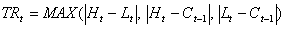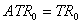Chart Pro online
User name (email):

 Recently used

 Forex

 Indices

# Average True Range (ATR)

Average True Range (ATR) is technical indicator which measures degree of price volatility. It was developed by J. Welles Wilder.

ATR is calculated by formula:First step in calculation of ATR is calculation of True Range (TR). True range is based on high and low values of current period (H and L) and close value of previous period (C). Second step is calculation of ATR using values of TR.

For ATR period is usually used value of 14.Average True Range (ATR)

Interpretation

Strong moves, in both directions, are often accompanied by large true ranges, especially at the beginning of move.

Welles Wilder found that high ATR values occur at market bottoms following a panic selling. On the other hand, low ATR values usually occur at market tops.

Example